# 车牌定位--颜色分割

+关注继续查看

1、车辆图像信息获取2、HSV颜色转换

hsvzation(image,hsv,width,height);

3、HSV颜色过滤

H值范围：190 ~ 245

S值范围： 0.35 ~ 1

V值范围： 0.3 ~ 14、噪声处理5、边缘检测6、确定车牌位置7、截取车牌图像1、读取复杂背景的车牌图像2、HSV滤波

HSV过滤后可以看到明显的干扰信息，车辆后面的栏杆，和车辆同样的颜色3、均值去噪4、边缘检测5,、 投影后错误的定位6、车牌提取错误1、HSV空间转换2、均值去噪3、水平膨胀4、边缘检测5、候选区域筛选1. int find_connected_region_location(struct BMP_img *img, unsigned char *src, int xthreashold, int ythreashold, float rateLow, float rateHigh)
2. {
3.     int i,j;
4.     int x1, y1, x2, y2;
5.     int width;
6.     int height;
7.     unsigned char *temp;
8.     //int queue_count;
10.     struct XY_Queue *queue;
11.     static int direction={{1, 0}, {-1, 0}, {0, 1}, {0, -1}};
12.
13.     width = img->width;
14.     height = img->height;
15.
16.     queue = (struct XY_Queue *)malloc(sizeof(struct XY_Queue) * width * height);
17.
18.     temp = (unsigned char *)malloc(width * height * sizeof(unsigned char));
19.
20.     if(temp == NULL)
21.     {
22.         printf("find_connected_region_location mem alloc fail\n");
23.         return -1;
24.     }
25.     memcpy(temp, src, width * height);
26.
27.     head = rear = 0;
28.     img->region_num = 0;
29.
30.     for(i = 0; i < height; i++)
31.         for(j = 0; j < width; j++)
32.     {
33.         if(temp[i * width + j] == 255)
34.         {
35.
36.             queue[rear].x = j;
37.             queue[rear].y = i;
38.             rear ++;
39.             temp[i * width + j] = 0;
40.
41.             img->pre_region[img->region_num].x1 = j;
42.             img->pre_region[img->region_num].x2 = j;
43.             img->pre_region[img->region_num].y1 = i;
44.             img->pre_region[img->region_num].y2 = i;
45.
46.             if(img->region_num > CAN_REGION_NUM)
47.             {
48.                 printf("over the CAN_REGION_NUM\n");
49.                 return -1;
50.             }
51.
53.             {
57.
58.                 if(x1 < img->pre_region[img->region_num].x1)
59.                     img->pre_region[img->region_num].x1 = x1;
60.                 else if(x1 > img->pre_region[img->region_num].x2)
61.                     img->pre_region[img->region_num].x2 = x1;
62.                 if(y1 < img->pre_region[img->region_num].y1)
63.                     img->pre_region[img->region_num].y1 = y1;
64.                 else if(y1 > img->pre_region[img->region_num].y2)
65.                     img->pre_region[img->region_num].y2 = y1;
66.
67.
68.                 for(i = 0; i < 4; i++)
69.                 {
70.                     x2 = x1 + direction[i];
71.                     y2 = y1 + direction[i];
72.
73.                     if(x2 > 0 && x2 < width && y2 > 0 && y2 < height && temp[y2 * width + x2])
74.                     {
75.                         temp[y2 * width + x2] = 0;
76.                         queue[rear].x = x2;
77.                         queue[rear].y = y2;
78.                         rear ++;
79.                     }
80.
81.                 }
82.             }
83.             if((img->pre_region[img->region_num].x2 - img->pre_region[img->region_num].x1 > xthreashold) && (img->pre_region[img->region_num].y2 - img->pre_region[img->region_num].y1 > ythreashold))
84.             {
85.
86.                 img->pre_region[img->region_num].width = img->pre_region[img->region_num].x2 - img->pre_region[img->region_num].x1 + 1;
87.                 img->pre_region[img->region_num].height = img->pre_region[img->region_num].y2 - img->pre_region[img->region_num].y1 + 1;
88.                 img->pre_region[img->region_num].rate = (float)img->pre_region[img->region_num].width/img->pre_region[img->region_num].height;
89.                 if((img->pre_region[img->region_num].width < img->width / 2) && (img->pre_region[img->region_num].height < img->height / 2))
90.                 if((img->pre_region[img->region_num].rate > rateLow) && (img->pre_region[img->region_num].rate < rateHigh))
91.                 {
92.                     if(img->pre_region[img->region_num].x2 + PRE_LOCATION_BIAS > img->width)
93.                             img->pre_region[img->region_num].x2 = img->width;
94.                     else
95.                             img->pre_region[img->region_num].x2 += PRE_LOCATION_BIAS;
96.                     if(img->pre_region[img->region_num].x1 - PRE_LOCATION_BIAS < 0)
97.                             img->pre_region[img->region_num].x1 = 0;
98.                     else
99.                             img->pre_region[img->region_num].x1 -= PRE_LOCATION_BIAS;
100.                     if(img->pre_region[img->region_num].y2 + PRE_LOCATION_BIAS > img->height)
101.                             img->pre_region[img->region_num].y2 = img->height;
102.                     else
103.                             img->pre_region[img->region_num].y2 += PRE_LOCATION_BIAS;
104.                     if(img->pre_region[img->region_num].y1 - PRE_LOCATION_BIAS < 0)
105.                             img->pre_region[img->region_num].y1 = 0;
106.                     else
107.                             img->pre_region[img->region_num].y1 -= PRE_LOCATION_BIAS;
108.
109.                     img->pre_region[img->region_num].width = img->pre_region[img->region_num].x2 - img->pre_region[img->region_num].x1 + 1;
110.                     img->pre_region[img->region_num].height = img->pre_region[img->region_num].y2 - img->pre_region[img->region_num].y1 + 1;
111.
112.                     img->region_num++;
113.                 }
114.             }
115.         }
116.
117.
118.     }
119.     free(temp);
120.     temp = NULL;
121.     return 0;
122. }

6、截取车牌区域图像1、车辆图像信息2、HSV过滤分割3、去噪4、 膨胀6、边缘检测7、候选区域 连通域筛选8、截取候选区域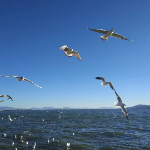NKMapView 显示标注，并放大到标注位置
NKMapView 显示标注，并放大到标注位置
12 0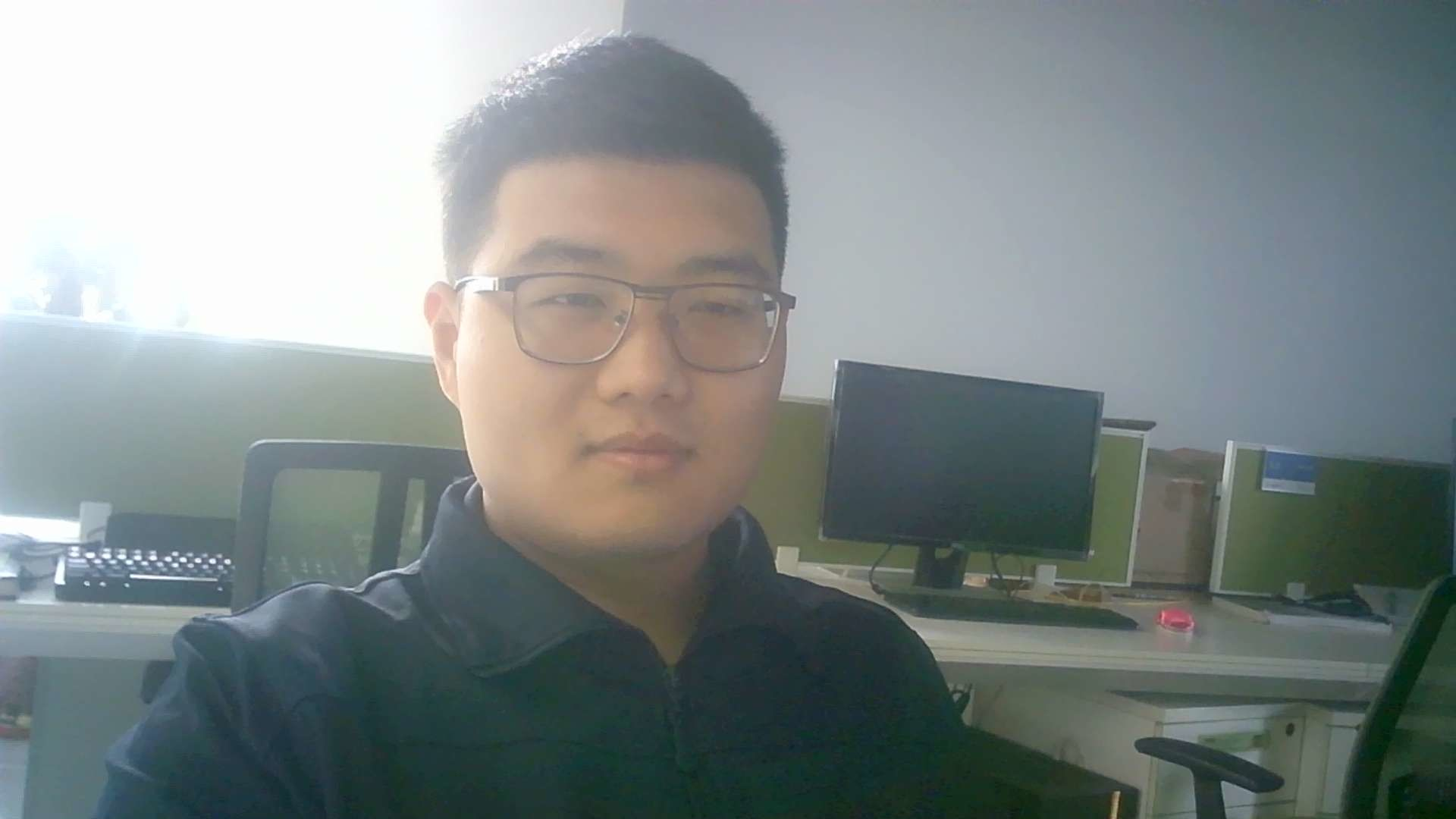156 0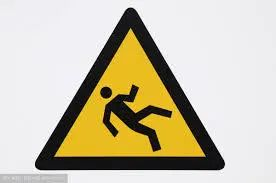Python + Opencv2 实现轮廓提取，轮廓区域面积计算； 对图像处理时，会遇到这样一个场景：找到图像主体轮廓，这是其一，可能为了凸显轮廓，需要用指定的颜色进行标记；轮廓标记完可能任务还没有结束，还需对轮廓所勾勒的像素面积区域统计计算。
849 0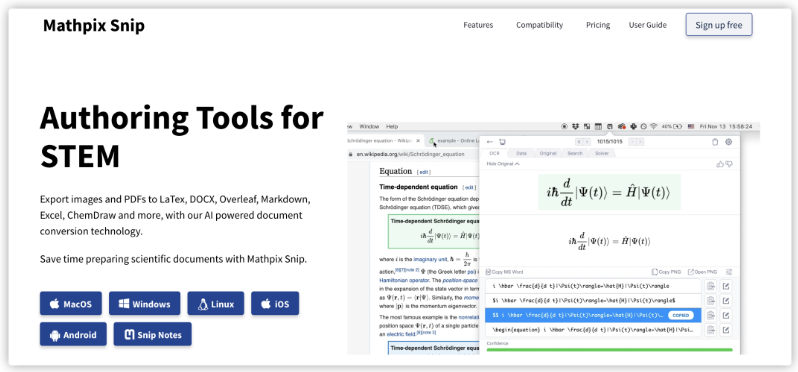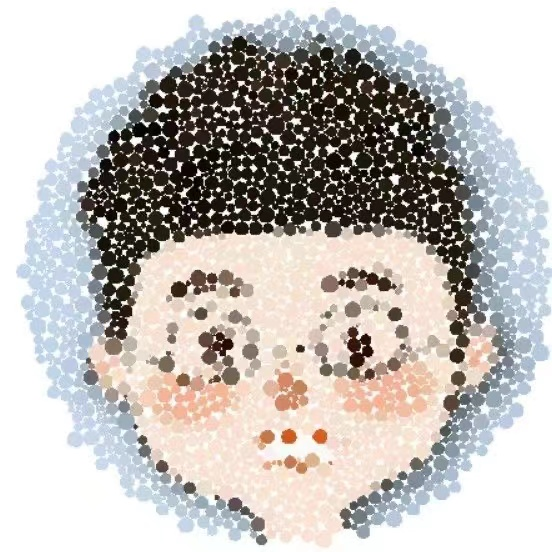Mathpix Snip除了识别公式，竟然还可以识别表格？

246 0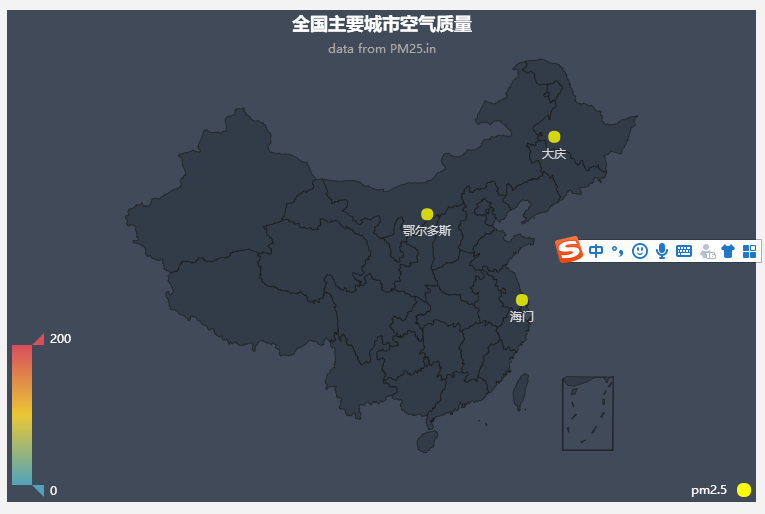100 0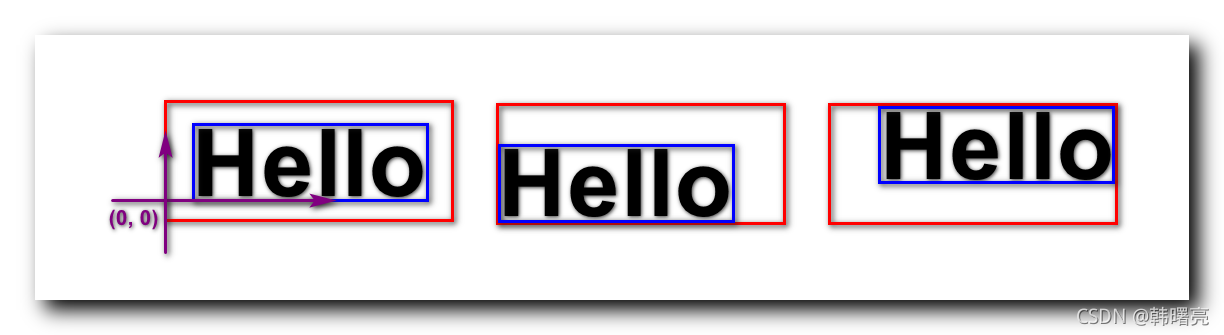【Android 应用开发】Canvas 精准绘制文字 ( 测量文本真实边界 | 将文本中心点与给定中心点对齐 )
【Android 应用开发】Canvas 精准绘制文字 ( 测量文本真实边界 | 将文本中心点与给定中心点对齐 )
77 0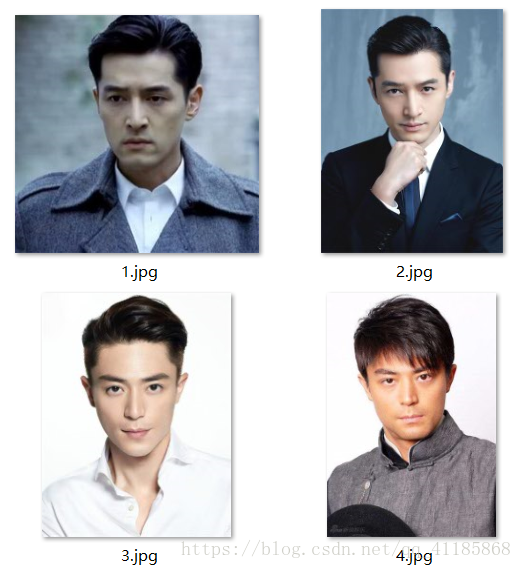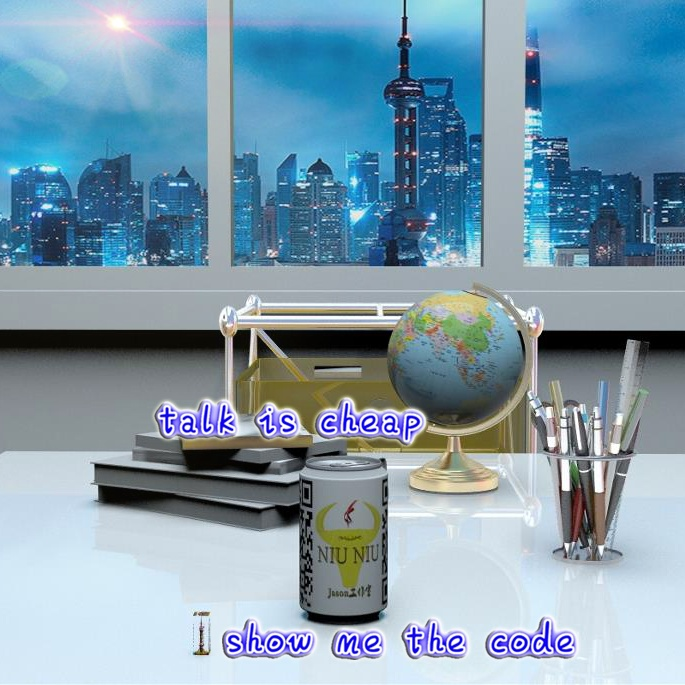CV之FR：DIY脚本通过人脸图像得到人脸特征向量并输出多张人脸图片之两两图片之间的距离
CV之FR：DIY脚本通过人脸图像得到人脸特征向量并输出多张人脸图片之两两图片之间的距离
64 0